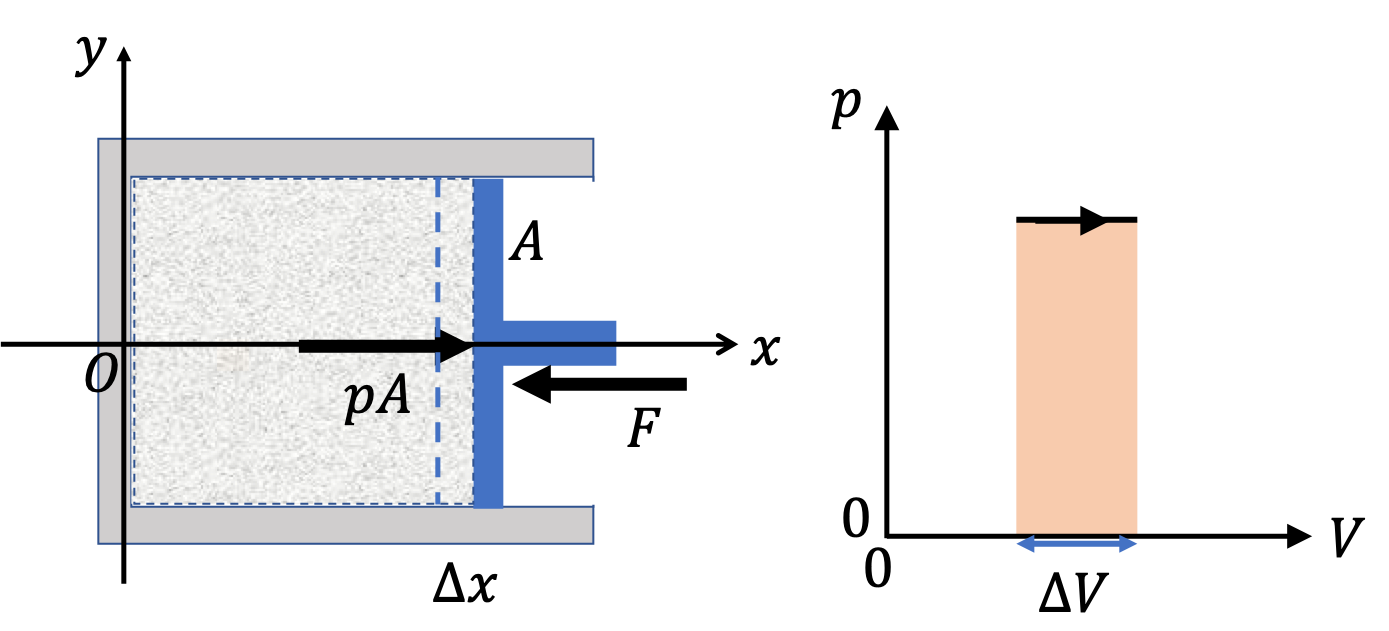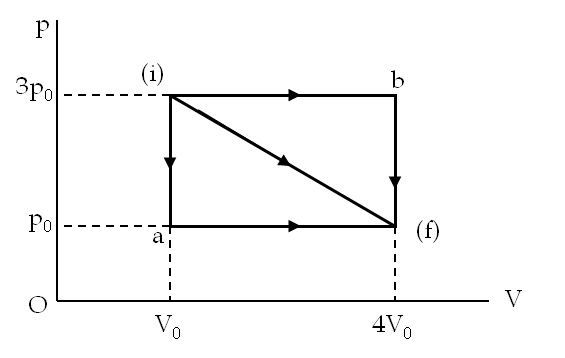## Section21.3Mechanical Work by Gas

### Subsection21.3.1Mechanical Work

Consider a system consisting of a fixed amount of a gas in a cylinder with a movable piston of area $A\text{.}$ Let pressure of the gas inside the system be $p\text{.}$ The piston is held in place by an external force $F$ that balances the push $pA$ by the gas molecules on the piston (Figure 21.3.1).

How much work will the gas do if the piston is pushed out by a distance $\Delta x\text{,}$ expanding the gas by a volume $\Delta V = A \Delta x\text{?}$ Work $\delta W$ by gas will be opposite of the work by the external force.

\begin{equation} \delta W_{\text{by gas}} = pA\Delta x = p\Delta V. \tag{21.3.1} \end{equation}

This is just the area in the $(p,V)$-plane. As a result of this work, energy of the gas will decrease by this amount.Figure 21.3.1. Calculating work done by a gas against an external agent applying a force $F\text{.}$ For a quasistatic process, the applied force $F$ on the piston is kept infintesimally less than the force by the gas so that the gas expands slowly and gently with pressure in gas kept at $p\text{.}$ The expansion process is shown in the horizontal line in $(p,V)$-plane. The work equals the area $p\Delta V$ under the $p$ line.

Figure 21.3.2 illustrates the work-from-area-under-the-curve in two situations - (a) an expansion, and (b) a cyclic process. A quasistatic process that leads to an expansion or compression of a material will be represented by a curve in the $(p,V)$-plane. The area under the curve gives us the total work by the gas - the expansion gives positive work and the compression negative work by the gas.Figure 21.3.2. (a) Mechanical work in a quasistatic process is the area under the $p$ versus $V$ curve of the process of expansion or contraction. (b) If the process is cyclic, it will contain both an expansion phase and a comntraction. The expansion will be positive work by the system and the contraction the negative work. The area enclosed in the cycle in the $(p,V)$-plane equals the net work in the cyclic process.
###### Remark21.3.3.Expanding Against Vacuum.

What happens if there is a vacuum outside the gas container and the container of the gas is punctured so that the gas can freely flow into the vacuum?

In this situation, the gas would not be in a mechanical equilibrium. Hence, you cannot assign any pressure to the entire gas. However, since, there would be no force opposing the movement of the gas molecules, the gas molecules would not do any work on any outside body. The molecules will, of course do work on each other if interactions between molecules are significant, which we will ignore for the moment.

\begin{equation} W_{if} = 0 \ \ \ \text{(expanding in vacuum.)}\tag{21.3.2} \end{equation}
###### Remark21.3.4.Expanding Into a Vacuum.

What if we keep gas in the container and let the piston move as gas expands against a vacuum?

The puncturing of the container to let the gas out into the vacuum is different from letting the piston of the gas cylinder move as a result of the pressure differential between inside and outside while keeping the gas inside the enclosed container. Suppose, you create vacuum in the space outside the cylinder of gas while keeping the piston fixed in place. Now, when you release the piston, there would be an imbalance of forces on the piston.

Let the mass of the piston be $M\text{.}$ Then, over the course of expansion of the gas by the amount $\Delta V\text{,}$ the piston would have moved $\Delta x$ under the force $pA\text{,}$ where $A$ is the area of cross-section of the cylinder. This would mean that gas would do the work on the piston of the amount $p\Delta V$ causing the piston to accelerate. The energy lost by the gas would go into changing the kinetic energy of the piston.

A gas in a cylinder undergoes change from an initial state i to a final state f shown in the figure through three paths, (a) i-a-f, (b) i-b-f, and (c) direct i-f. Find work done by the gas for each path.Hint

Use area under $p$ versus $V$ curve to find the work.

(a) $3 p_0 V_0\text{,}$ (b) $9 p_0 V_0\text{,}$ (c) $6p_0V_0\text{.}$

Solution

(a) Work on path i-a-f:

This path has two segments: i-a and a-f. On segment i-a, the volume $V$ does not change, therefore $W_{\text{i-a}} =0\text{.}$ On the segment a-f, the pressure is constant, therefore, the area under the curve is just a product of the constant pressure and the change in volume. Therefore, work done by the gas

\begin{equation*} W_{\text{i-a-f}} = p_0\times(4V_0 - V_0) = 3 p_0 V_0. \end{equation*}

(b) Work on path i-b-f:

This path has two segments: i-b and b-f. On segment i-b, the pressure is constant with value $3p_0$ and the volume changes from $V_0$ to $4V_0\text{.}$ Therefore,

\begin{equation*} W_{\text{i-b}} = 3p_0 \times 3 V_0 = 9 p_0 V_0. \end{equation*}

The work done on segment b-f is zero since the volume is constant during this process. Therefore, work done by the gas

\begin{equation*} W_{\text{i-b-f}} = 9 p_0 V_0. \end{equation*}

Make note that this is different than what happened on path i-a-f.

(c) Work on direct path i-f:

The area under the curve is that of a rectangle and a triangle. Since the process is an expansion, work by the gas will be positive.

\begin{equation*} W_{\text{i-f}} = \left( p_0\times 3V_0 +\frac{1}{2}\times 2p_0\times 3V_0 \right)= 6p_0V_0. \end{equation*}

### Subsection21.3.2(Calculus) Mechanical Work by Ideal Gas

Consider a system consisting of a fixed amount of an ideal gas in a cylinder with a movable piston of area $A$ as in Figure 21.3.1. We now look at work in an infinitesimal expansion, corresponding to the distance $dx$ moved by the piston. Work $\delta W$ by gas will be opposite of the work by the external force.

\begin{equation} \delta W_{\text{by gas}} = pA dx = p dV, \tag{21.3.3} \end{equation}

where $dV = A dx\text{.}$ If gas expands so that its thermodynamic state changes from $(p_i,\, V_i,\, T_i)$ to $(p_f,\, V_f,\, T_f)\text{,}$ we need to sum up work on every infinitesimal change.

\begin{equation*} W_{if} = \int_{i}^{f}\, p dV. \end{equation*}

For an ideal gas, we have $p = nRT/V\text{.}$ This gives

\begin{equation} W_{if} = nR\int_{i}^{f}\, \dfrac{T}{V} dV.\label{eq-work-by-ideal-gas}\tag{21.3.4} \end{equation}

Here $T$ will also change with $V$ depending on how the change was accomplished, whether the process was isothermal or adiabatic or something else.

#### Subsubsection21.3.2.1(Calculus) Work by Gas in an Isothermal Process

In an isothermal process temperature does not change. Therefore, in Eq. (21.3.4), the temperature will come out of integral, and then the integration can be easily performed.

\begin{equation} W_{if} = nRT\int_{i}^{f}\, \dfrac{1}{V} dV = nRT\ln\left( \dfrac{V_f}{V_i}\right).\tag{21.3.5} \end{equation}

#### Subsubsection21.3.2.2(Calculus) Work by Gas in an Adiabatic Process

When a gas expands it loses energy to the environment, consequenctly, the gas cools. In a quasi-static adiabatic process of an ideal gas, pressure and volume change so that

\begin{equation*} pV^{\gamma} = \text{constant}, \end{equation*}

where $\gamma$ is ratio of specific heats, namely, $\gamma=c_P/c_V\text{,}$ where $c_P$ is specific heat at constant pressure and $c_V$ specific heat at constant volume; specific heat is the amount of energy you need to change the temperature of $1\text{ kg}$ of gthe sample by one degree. For monatomic gas, $\gamma=5/3\text{,}$ and for diatomic gas, $\gamma=7/5\text{.}$ Using this as giving us function $p(V)$ on the path in the $(p,V)$-plane,

\begin{equation*} pV^\gamma = p_i V_i^\gamma, \end{equation*}

we can express the work integral as:

\begin{equation*} W_{if} = \int_{i}^{f}\, p dV = p_iV_i^{\gamma}\int_{i}^{f}\, \dfrac{dV}{V^{\gamma}}. \end{equation*}

Since $\gamma\ne 1$ we will get

\begin{equation*} W_{if}^{\text{adiabatic}} = \dfrac{p_iV_i^{\gamma}}{1-\gamma}\left( V_f^{1-\gamma} - V_i^{1-\gamma} \right), \end{equation*}

which simplifies by using $p_iV_i^{\gamma} = p_fV_f^{\gamma}$ to be

\begin{equation} W_{if}^{\text{adiabatic}} = \dfrac{1}{1-\gamma} \left( p_fV_f - p_i V_i\right).\tag{21.3.6} \end{equation}

Oxygen gas in a container is compressed along a path that can be described by the following pressure $p$ in atm as a function of volume $V\text{,}$ with $V_0 = 1\text{ L}\text{.}$

\begin{equation*} p=(3.0\ \text{atm})\left(V/V_0\right)^{-1.2}. \end{equation*}

The initial and final volumes during the process were $2\text{ L}$ and $1.5\text{ L}$ respectively. Find the amount of work done on the gas.

Hint

Integrate with a minus sign.

$0.77 \ \text{L.atm} = 78\text{ J}\text{.}$

Solution

Here the work required is the work on the gas. Therefore, we will calculate the integral with the minus sign.

\begin{equation*} W_\text{on} = - \int p dV. \end{equation*}

The integral for the given function gives

\begin{align*} W_\text{on} \amp = - \int_{V_1}^{V_2} p dV = - \frac{3.0\ \text{atm} V_0^{1.2}}{-0.2}\left[ \frac{1}{V_2^{0.2}} - \frac{1}{V_1^{0.2}} \right]. \end{align*}

Now, we put in the numerical values of $V_0$, $V_1$ and $V_2$ to obtain

\begin{gather*} W_\text{on} = -\frac{3.0\ \text{atm} (1\ \text{L})^{1.2}}{-0.2} \left[ \frac{1}{(1.5\ \text{L})^{0.2}} - \frac{1}{(2\ \text{L})^{0.2}} \right] = 0.77 \ \text{L.atm} \end{gather*}

Check if the sign is correct - since contraction of the gas, we expect a positive sign for the work on the gas as found. In terms of $\text{J}$ the answer would be $78\text{ J}\text{.}$

A cylinder containing three moles of a monatomic ideal gas is heated at a constant pressure of $2 \text{ atm}\text{.}$ The temperature of the gas changes from $300\text{K}$ to $350\text{K}$ as a result of the expansion. Find work done (a) on the gas, and (b) by the gas.

Hint

Get $V_i$ and $V_f$ from using ideal gas law and use them in the integral.

(a) $1245\text{ J}\text{,}$ (b) $-1245\text{ J}\text{.}$

Solution

(a) Since the pressure of the gas is constant during the change, the work integral will simply equal the product of the pressure and the change in the volume.

\begin{equation*} W = \int p dV = p \Delta V. \end{equation*}

So, we need to find the change in the volume to plug into this formula. From the given data we can find the volumes at the two ends of the process using the ideal gas law for those states.

\begin{align*} \amp V_i = \frac{nR}{p} T_i\\ \amp V_f = \frac{nR}{p} T_f \end{align*}

Therefore, the change in the volume is

\begin{equation*} \Delta V = V_f - V_i = \frac{nR}{p} \left( T_f -T_i\right). \end{equation*}

Now putting this in the work we get work by the gas to be

\begin{equation*} W = p\Delta V = nR \left( T_f -T_i\right). \end{equation*}

By carrying out the calculation symbolically we find that the pressure cancels out. This reduces the actual numerical work we need to do.

\begin{align*} W_{by} \amp = 3\ \text{mol}\times 0.082\frac{\text{L.atm}}{\text{mol.K}}\times \left( 350 -300\right)\ \text{K}\\ \amp = 12.3 \ \text{L.atm} = 1250\ \text{J}. \end{align*}

(b) Work done by the gas will have the same magnitude as done on the gas but opposite in sign. therefore, we will get $-1250\ \text{J}\text{.}$